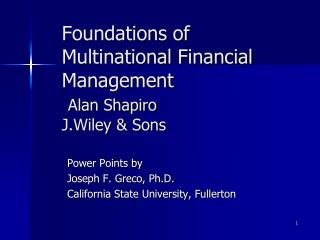# Foundations of Multinational Financial Management Alan Shapiro J.Wiley & Sons - PowerPoint PPT PresentationDownload PresentationFoundations of Multinational Financial Management Alan Shapiro J.Wiley & Sons

Foundations of Multinational Financial Management Alan Shapiro J.Wiley & SonsDownload Presentation## Foundations of Multinational Financial Management Alan Shapiro J.Wiley & Sons

- - - - - - - - - - - - - - - - - - - - - - - - - - - E N D - - - - - - - - - - - - - - - - - - - - - - - - - - -
##### Presentation Transcript

1. Foundations of Multinational Financial ManagementAlan ShapiroJ.Wiley & Sons Power Points by Joseph F. Greco, Ph.D. California State University, Fullerton

2. The Determination of Exchange Rates Chapter 2

3. CHAPTER 2THE DETERMINATION OF EXCHANGE RATES CHAPTER OVERVIEW: I. EQUILIBRIUM EXCHANGE RATES II. ROLE OF CENTRAL BANKS III. EXPECTATIONS AND THE ASSET MARKET MODEL

4. Commonly Used Terms • Pegged Currency • Devaluation • Revaluation • Floating currency • Depreciation • Appreciation

5. Part I. Equilibrium Exchange Rates I. SETTING THE EQUILIBRIUM A. The exchange rate is the local currency price of one unit of foreign currency For example \$1.30/€ means the euro in theU.S. is worth \$1.30.

6. The Demand for € in the U.S. B. How Americans Purchase German Goods 1. Foreign Currency Demand -derived from the demand for a foreign country’s goods, services, and financial assets. e.g. The demand for euros comes from the demand for German goods by Americans

7. The Demand for € in the U.S. \$/€ D \$.50 Qty

8. Equilibrium Exchange Rates B.2. Foreign Currency Supply: a. derived from the foreign country’s demand for local goods.

9. b. They must convert their currency to purchase the foreign goods. That means the supply of euros comes from the German demand for US goods which means Germans convert euros to US \$ in order to buy.

10. The Supply of € in the U.S. \$/ € S \$.50 Qty

11. Equilibrium Exchange Rates B.3. Equilibrium Exchange Rate occurs where the quantity supplied equals the quantity demanded of a foreign currency at a specific local price.

12. The \$/ € Equilibrium Rate \$/ € Equilibrium D S \$.50 Qty

13. Equilibrium Exchange Rates C. How Exchange Rates Change 1. Increased demand as more foreign goods are demanded, more of the foreign currency is demanded at each possible exchange rate 2. The price of the foreign currency in local currency increases.

14. Equilibrium Exchange Rates C.3. Home Currency Depreciation a. Foreign currency more valuable than the home currency. b. Conversely, then the foreign currency’s value has appreciated against the home currency.

15. The US\$ Depreciates When \$/ € D’ D \$.65 S \$.50 Qty Q1 Q2

16. Rules of Calculation If Numerator currency depreciates, e1 > e0:\$0.50 (e0) to \$0.65 (e1), then calculate % depreciation by using = (e0 - e1)/ e1 And Denominator currency appreciates, then calculate % appreciation by using = (e1 - e0)/ e0

17. Equilibrium Exchange Rates C.5 Currency Appreciation = (e1 - e0)/ e0 where e0 = old currency value e1 = new currency value

18. Equilibrium Exchange Rates EXAMPLE: € Appreciation If the dollar value of the € goes from \$0.50 (e0) to \$0.65 (e1), then the € has appreciated by (.65 - .50)/ .50 = 30%

19. Equilibrium Exchange Rates C.4. Calculating a Depreciation: = (e0 - e1)/ e1 where e0 = old currency value e1 = new currency value

20. Equilibrium Exchange Rates EXAMPLE: US\$ Depreciation Use the formula (e0 - e1)/ e1 substituting (.50 - .65)/ .65 = - 23.1% is the US\$ depreciation

21. COMPUTATION GUIDELINES If you are given a rate of appreciation or depreciation and asked to find the opposite value: Given: Find: or

22. Sample Problem No.1 Suppose the U.S. dollar appreciates against the Russian ruble by 500%. How much did the ruble depreciate against the dollar?

23. U.S. \$ APPRECIATION

24. Depreciation of the ruble:

25. SOLUTION

26. When the dollar appreciated by 500% against the ruble, the ruble depreciated 83% against the dollar.

27. Sample Problem No.2 • Suppose the Russian ruble depreciates against the U.S. dollar by 83%. How much did the dollar appreciates against the ruble?

28. Depreciation of the ruble:

29. U.S. \$ APPRECIATION

30. SOLUTION

31. Find the appreciation: Substituting: Summing the numerator

32. Equilibrium Exchange Rates D. OTHE FACTORS AFFECTING EXCHANGE RATES: 1. Relative Inflation rates 2. Relative Interest rates 3. Relative economic growth rates 4. Political risk 5. Expectations